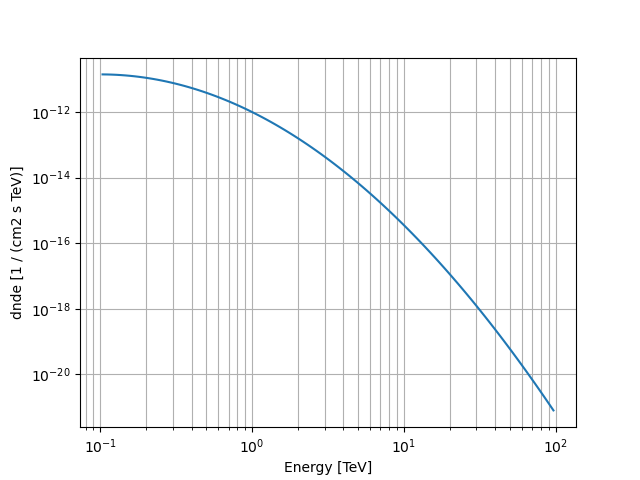# Log parabola spectral model#

This model parametrises a log parabola spectrum.

It is defined by the following equation:

$\phi(E) = \phi_0 \left( \frac{E}{E_0} \right) ^ { - \alpha - \beta \log{ \left( \frac{E}{E_0} \right) } }$

Note that $$log$$ refers to the natural logarithm. This is consistent with the Fermi Science Tools and ctools. The Sherpa package, however, uses $$log_{10}$$. If you have parametrization based on $$log_{10}$$ you can use the from_log10() method.

## Example plot#

Here is an example plot of the model:

from astropy import units as u
import matplotlib.pyplot as plt
from gammapy.modeling.models import LogParabolaSpectralModel, Models, SkyModel

energy_bounds = [0.1, 100] * u.TeV
model = LogParabolaSpectralModel(
alpha=2.3,
amplitude="1e-12 cm-2 s-1 TeV-1",
reference=1 * u.TeV,
beta=0.5,
)
model.plot(energy_bounds)
plt.grid(which="both")## YAML representation#

Here is an example YAML file using the model:

model = SkyModel(spectral_model=model, name="log-parabola-model")
models = Models([model])

print(models.to_yaml())


Out:

components:
-   name: log-parabola-model
type: SkyModel
spectral:
type: LogParabolaSpectralModel
parameters:
-   name: amplitude
value: 1.0e-12
unit: cm-2 s-1 TeV-1
-   name: reference
value: 1.0
unit: TeV
frozen: true
-   name: alpha
value: 2.3
-   name: beta
value: 0.5


Gallery generated by Sphinx-Gallery